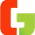## PEMDAS calculator for solving math questions:

PEMDAS calculator is able to solve math questions that may involve 2 or more operators including multiplication, division, addition, subtraction, parenthesis & exponents. We can solve these questions by hand too using PEMDAS rule.

### What is PEMDAS & why it being important in solving math questions?

PEMDAS stands for parenthesis, exponent, multiplication, division, addition & subtraction. It can be used for solving 2 or more operations in a given math expression, in order of letters in PEMDAS. This rule tells you to solve parenthesis first, followed by exponent. Then comes multiplication & division, but they have same priority so we need to solve operation from left to right. Then we have addition & subtraction that too have same priority so the order of operation is from left to right.

### Examples:

#### Question 1: 16 / 4 (2 + 3) = ?

We solve parenthesis first,
= 16 / 4 x (2 + 3)
= 16 / 4 x (5)
= 16 / 4 x 5

Division & multiplication have same priority, so the order of operation is from left to right.
= 16 / 4 x 5
= 4 x 5
= 20

#### Question 2: 2^2 + 5 x 4 = ?

First we solve exponent that have the highest priority
= 2^2 + 5 x 4
= 4 + 5 x 4

Multiplication has higher priority than addition:
= 4 + 20
= 24

If you find calculation difficult then use PEMDAS calculator that can be used for scientific purposes too. Order of operations calculator has everything required for solving math problems.

Order of Operations Calculator

1.1.